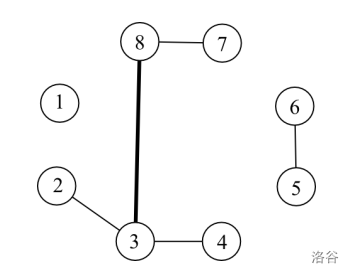/ FtOJ / 比赛 / 测试 /

# Description# Format

## Input

• A x y：表示在 $$x$$ 和 $$y$$ 之间连一条边。保证之前 $$x$$ 和 $$y$$ 是不联通的。
• Q x y：表示询问 $$(x,y)$$ 这条边上的负载。保证 $$x$$ 和 $$y$$ 之间有一条边。

# Sample 1

## Input

8 6
A 2 3
A 3 4
A 3 8
A 8 7
A 6 5
Q 3 8


## Output

6


1s, 125MB.

BJOI 2014

ACM/ICPC

1

2020-05-10 19:30

2020-05-10 22:30

3.0 小时

1